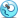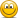# Exam FeedbackFRM Part 1 (November 2014) Exam Feedback

##### New Member
Sorry, the answer said just key rate, because if it had said key rate 01, I would have chosen Duration then.
was it key rate, key rate'01 or key rate duration? "key rate" itself does not mean key rate duration and the changes of share prices following changes in interest rate. I picked Duration as well, do you remember what more information that pointed to key rate?

#### hamu4ok

##### Active Member
was it key rate, key rate'01 or key rate duration? "key rate" itself does not mean key rate duration and the changes of share prices following changes in interest rate. I picked Duration as well, do you remember what more information that pointed to key rate?
I clearly remeber it was just key rate, but I dont' remember the wording of the question now. I admit I might have incorectly chosen key rate. First, I opted for duration, but the qestion seemed to me have twist.

##### New Member
I was rushing through this question, could even remember not reading the whole question properly.... If it actually asked about "rate of change"... the answer would be convexity then...

#### LMFRM

##### Member
Subscriber
With regards to a question about an analyst backtesting a 99% VaR, and to calculate the probablity of 2 exceedences:

I finally figured this out at my desk. The key is to use the binomial equation where probability of exceedence, p = 0.01 and X = exceedence

P(X=2) = 90C2 x (0.01)^2 x (1 - 0.01)^88 = 16.54% (Answer A)

By the roll of an imaginary die, I managed to answer this correctly. It looks like one of those questions where GARP decided to take two AIMs in different sections and combine it into one question to test candidates.

Hi,
I didn't succeed to compute the '90!' with my TI BA II +, I got an 'Error', is someone has the same problem?

#### LMFRM

##### Member
Subscriber
Yes, I mean the same. It was a question 77 I think. It was asked which of the measures did not use benchmark or market index for their calculations? And in the answer were: Information, Treynor, Sharpe and Sortino. Because only Information ratio use return od the benchmark, I think something was wrong here.

Hi,
I think, the answers were Information, Treynor, Sharpe et Jensen's alpha, I don't think Sortino was in the answers.

#### JDGutzmann

##### Member
Hi,
I didn't succeed to compute the '90!' with my TI BA II +, I got an 'Error', is someone has the same problem?

Hi, none of the calculators do this, it was possible if you mentally wrote all the multipliers down and cancelled out most of them:

numerator.: n! : (1*2*3*...*87*88*89*90)
denominator: (n-k)! k! : (1*2*3*...*87*88) * (1*2)

all the underlined figures cancel out so you are left with 89*90/2, then the rest of the binomial equation follows.

Hope, this helps

#### LMFRM

##### Member
Subscriber
Hi, none of the calculators do this, it was possible if you mentally wrote all the multipliers down and cancelled out most of them:

numerator.: n! : (1*2*3*...*87*88*89*90)
denominator: (n-k)! k! : (1*2*3*...*87*88) * (1*2)

all the underlined figures cancel out so you are left with 89*90/2, then the rest of the binomial equation follows.

Hope, this helps

Thank u

#### ShaktiRathore

##### Well-Known Member
Subscriber
Hi,
I didn't succeed to compute the '90!' with my TI BA II +, I got an 'Error', is someone has the same problem?
In TI BA II i think u can calculate 90C2 with nCn function given so whats the problem.it may ve a problem for hp.
Thanks

#### LucasC

##### New Member
There was a linear regression question that provided a scattered plot with heteroskedasticity. I ruled out 2 answer choices and was left with BLUE and asymptotic normality. Because of the heteroskedasticity I ruled out BLUE as well, but I don't remember seeing this concept of asymptotic normality in any of the review notes I had gone through. Was this concept mentioned anywhere in the notes?

#### Tural Hasanov

##### New Member
There was a linear regression question that provided a scattered plot with heteroskedasticity. I ruled out 2 answer choices and was left with BLUE and asymptotic normality. Because of the heteroskedasticity I ruled out BLUE as well, but I don't remember seeing this concept of asymptotic normality in any of the review notes I had gone through. Was this concept mentioned anywhere in the notes?
Stock, Chapter 5: Single Regression: Hypothesis Tests and Confidence Intervals study notes chapter end 5th question in bionicturtle.
Both these answers in the question are true (question asks for the wrong one):
a)Whether the errors are homo- or heteroskedastic, the OLS estimators are are unbiased, consistent and asymptotically normal
c)If it is true that, in addition to the three assumptions above, that the errors are homoskedastic, then our OLS estimator for B(1) is BLUE

By the way, I don't remember the question in frm exam exactly but I remember the answer that the coefficients are asymptotically normal (A in frm question). If there is heteroscedasticity in the model then the OLS estimators are not efficient (do not have least variance) therefore I think they are not BLUE.

#### berimbolo

##### New Member
Subscriber
There was an answer with consistent and unbiased, that didn't contain efficient.

#### LucasC

##### New Member
There was an answer with consistent and unbiased, that didn't contain efficient.
I was choosing between A (asymptotic normality) and D(BLUE) and ruled out BLUE to go for A. I don't remember this answer.
So if “Whether the errors are homoskedastic or heteroskedastic, the OLS estimator is unbiased, consistent, and asymptotically normal.”, then which is the correct one...

#### berimbolo

##### New Member
Subscriber
I was choosing between A (asymptotic normality) and D(BLUE) and ruled out BLUE to go for A. I don't remember this answer.
So if “Whether the errors are homoskedastic or heteroskedastic, the OLS estimator is unbiased, consistent, and asymptotically normal.”, then which is the correct one...

I think you are correct, it should be A.

#### Raghavsrini

##### New Member
Hi guys, I am new to BT... as the result day approaches, I am quite nervous about how my Part I (November 2014) went... I personally felt that the exam was quite tough, given that I prepared hard for it... I hope there are people who felt the same way I did.. Please lemme know if the difficulty level of the exam was on the higher side in November or if it is the typical difficulty level of exam... I hope the pass rate is above 45%..

#### SRayan

##### New Member
Now I completely forgot how i performed in the exam....I would say the chances are 50/50.... Just fingers crossed.

#### sandeep erramilli

##### New Member
Subscriber
at what time results will be released

#### VishalJ

##### New Member
Subscriber
Hi Sandeep, I read somewhere that the results will be released at 4PM EST cant find the post now.

#### Tipo

##### Member
Subscriber
Hi Sandeep, I read somewhere that the results will be released at 4PM EST cant find the post now.
Another 13 hrs to go i guess#### Pistakkio

##### New Member
I can see the results!!

Last edited:

#### VishalJ

##### New Member
Subscriber
Hey guys results are out..ALL the best

Replies
22
Views
3K
Replies
36
Views
5K
Replies
338
Views
84K
Replies
57
Views
18K
Replies
44
Views
11K